Subject 7. Demand Elasticities
#cfa #cfa-level-1 #economics #has-images #microeconomics #reading-13-demand-and-supply-analysis-introduction
Elasticity means "responsiveness." The elasticity of demand measures the responsiveness of the quantity demanded of a product to changes in any of the factors that affect demand. Analysts are interested in knowing how much the quantity demanded will rise or fall for a given change in price or income.

Price elasticity of demand is the percentage change in the quantity of a product demanded divided by the percentage change in the price causing the change in quantity. It indicates the degree of consumer response to variation in price. Specifically, it tells the analyst the percentage change in the quantity demanded for a good caused by a 1% increase in the price of that good.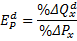The change in price is expressed as a percentage of the average price - the average of the initial and new price, and the change in the quantity demanded is expressed as a percentage of the average quantity demanded - the average of the initial and new quantity. Using the average price and average quantity, the same elasticity value is obtained regardless of whether the price rises or falls.

The measure is units-free because it is a ratio of two percentage changes and the percentages cancel each other out. Changing the units of measurement of price or quantity leave the elasticity value the same.

Because a change in price causes the quantity demanded to change in the opposite direction, this ratio is always negative, although economists always ignore the sign and simply use the absolute value. It is the magnitude, or absolute value, of the measure that reveals how responsive the quantity change has been to a price change.

Example 1

A Pizza Hut store can sell 50 pizzas per day at $7 each or 70 pizzas per day at$6 each. The price elasticity is: [(50 - 70)/60] / [(7 - 6) / 6.5] = -2.17.

Own-Price Elasticity of Demand

Demand can be inelastic, unit elastic, or elastic, and can range from zero to infinity. (Note: the negative sign is ignored.)

• If the elasticity coefficient is greater than 1, demand is elastic. A small price change leads to a large change in the quantity demanded. The more elastic the demand, the flatter the demand curve over any specific range. Demands for goods with many substitutes (e.g., juice) are relatively elastic. If the demand curve of a good is completely horizontal, the demand is perfectly elastic. Consumers will buy all of that good at the market price.
• When the elasticity coefficient is less than 1, demand is inelastic. The more inelastic the demand, the steeper the demand curve. Demands for goods with few substitutes (e.g., cigarettes) are relatively inelastic.
• When the elasticity coefficient is equal to 1, demand is said to be unitary elastic.

Because elasticity is a relative concept, the elasticity of a straight-line demand curve will differ at each point along the demand curve. Specifically, a straight-line demand curve is more elastic when price is high. Note that the elasticity is not the slope of the demand curve. Elasticity is used since it is independent of the units of measure.

Example 2

Refer to the graph below. Which of the following is true?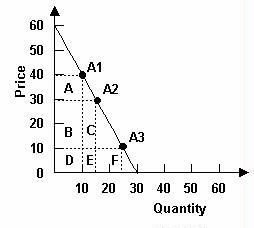A. Areas C and E are smaller than area A, so demand must be elastic between $10 and$30.
B. Areas C and E are smaller than area A, so demand must be inelastic between $10 and$30.
C. Area F is smaller than areas B and C, so demand must be inelastic between $10 and$30.

Answer: C. Since at $30 the demand is unit elastic, at prices below$30 demand is inelastic. This is because when price rises from $10 to$30, the revenue gained is greater than the revenue lost.

The Factors that Influence the Elasticity of Demand

The elasticity of demand among products varies substantially. The determinants of price and income elasticity of demand are:

• The closeness of substitutes. The most important determinant is the availability of substitutes. The closer the substitutes for a good or service, the more elastic the demand for it.

• Necessities, such as food or housing, generally have inelastic demand.
• Luxuries, such as exotic vacations, generally have elastic demand.

When good substitutes for a product are available, a price rise induces many consumers to switch to other products. For example, when the price of apples rises, many consumers simply switch to oranges or other fruits. However, when the price of gasoline rises, most consumers can only slightly cut back their consumption of gasoline, since there is no good substitute for gasoline.

• The proportion of income spent on the good. If expenditures on a product are quite small relative to a consumer's budget, the income effect will be small even if there is a substantial increase in the price of the product. This will make the demand less elastic. For example, if the price of matches triples, consumers would not bother to find substitutes, since they only spend a few bucks on matches each year.

• The time elapsed since a price change. The more time consumers have to adjust to a price change, or the longer a good can be stored without losing its value, the more elastic the demand for that good. In general, when the price of a product increases, consumers will reduce their consumption by a larger amount in the long run than in the short-run. Therefore, the demand for most products will be more elastic in the long run than in the short run.

The price elasticity of demand tends to increase in the long run.

As changing market conditions raise or lower the price of a product, both consumers and producers will respond. However, their response will not be instantaneous, and it is likely to become larger over time. In general, when the price of a product increases, consumers will reduce their consumption by a larger amount in the long run than in the short run. Thus, the demand for most products will be more elastic in the long run than in the short run. This relationship between the elasticity coefficient and the length of the adjustment period is referred to as the second law of demand.

Impact on Total Expenditure

Consumers' total expenditure is the same as total revenues from the suppliers' point of view. One of the most important applications of price elasticity is determining how total consumer expenditure on a product changes when the price changes.

Total Revenues = Total Expenditures = Price x Quantity

According to the law of demand, price and quantity move in opposite directions. When the price changes, total revenue also changes. But a rise in price doesn't always increase total revenue. The change in total expenditures depends on whether the effect of the changes in price or the effect of the changes in quantity is greater.

• When demand is inelastic, a change in price will cause total expenditures to change in the same direction.
• When demand is elastic, a change in price will cause total expenditures to move in the opposite direction.
• When demand elasticity is unitary, total expenditures will remain unchanged as price changes.

Because of the relationship between price and quantity sold, a firm's total revenue can rise, fall or stay the same in response to a change in price. The outcome is determined by the price elasticity of demand. This conclusion is similar to that of total expenditures.

Note that firms attempt to maximize profit (total revenue minus total cost), not revenue.

Income Elasticity of Demand: Normal and Inferior Goods

Definition: The percentage change in the quantity of a product demanded divided by the percentage change in consumer income causing the change in quantity demanded.

Since increases in consumer income will increase the demand for most goods, income elasticity measures the responsiveness of a demand for a good to a change in income. Specifically, it tells the analyst the percentage change in the quantity demanded for a good caused by a 1% increase in consumer income.

Calculation: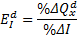The type of product is the primary determinant of income elasticity of demand.

• Most products have positive income elasticity; normal goods have positive income elasticity.

• Necessities have low income elasticities (between 0 and 1); when income rises by 1%, the quantity demanded for necessities will increase by less than 1%.
• Luxuries have high income elasticities (greater than 1).

• A few commodities (inferior goods) have negative income elasticity; as income expands, the demand for them will decline. Examples of inferior goods are margarine, junk food, etc.

Cross-Price Elasticity of Demand: Substitutes and Complements

The cross elasticity of demand is a measure of the responsiveness of demand for a good to a change in the price of a substitute or a complement, other factors remaining the same. The formula for calculating the cross elasticity is: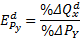• The cross elasticity of demand for a substitute is positive.
• The cross elasticity of demand for a complement is negative.

The following figure shows the increase in the quantity of pizza demanded when the price of a burger (a substitute for pizza) rises. The figure also shows the decrease in the quantity of pizza demanded when the price of a soft drink (a complement of pizza) rises.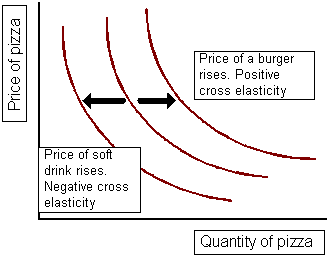If you want to change selection, open original toplevel document below and click on "Move attachment"